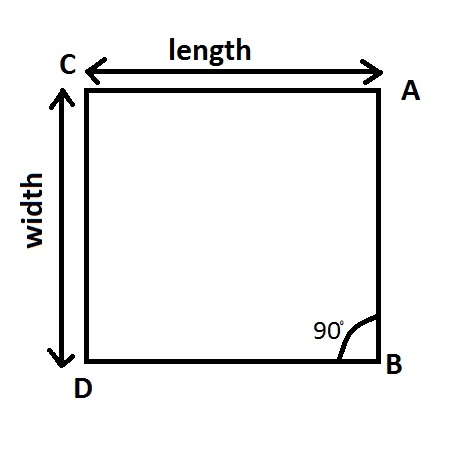# A page should have perimeter of 42 inches. The printing area within the page would be determined...

## Question:

A page should have perimeter of {eq}42 {/eq} inches. The printing area within the page would be determined by top and bottom margins of {eq}1 {/eq} inch from each side, and the left and right margins of {eq}1.5 {/eq} inches from each side. What should be the overall dimensions of the page in order to maximize the printing area?

## Rectangle

A rectangle is a two dimensional figure with two pair of equal straight sides that are all at right angles, i,e. {eq}90^o. {/eq}

The image shown below is a figure of rectangle ABCDFollowing are the properties of a rectangle:

Area: {eq}length * width {/eq}

Perimeter: {eq}2* (length + width) {/eq}

Number of Sides: {eq}4 {/eq}

Number of edges: {eq}4 {/eq}

Sum of angles : {eq}360^o {/eq}

Let the length and width of the page is {eq}x {/eq} and {eq}y. {/eq}

The perimeter of the page is 42. $$Perimeter= 2(Length + Width)$$

$$42 = 2(x + y)$$

Dividing the equation by 2

$$21= x + y$$

The length of the page is reduced by 1 inches from both margins and the width is reduced by 1.5 inches from width from both margin.

The new dimension of the printing area is: $$(x-2) \times (y-3)$$

the area of the printing area: $$Area_p= Length \times Width$$

$$Area_p= (x-2) \times (y-3)$$

To get the maximum area of a rectangle we need to make it a square or as close to it as possible.

Taking {eq}x = 10 {/eq} and {eq}y = 11 {/eq}

$$Area_p= (x-2) \times (y-3)$$

$$Area_p= (10-2) \times (11-3)$$

$$Area_p= 8 \times 8$$

$$Area_p= 64\ inches^2$$

Area of the printable page is {eq}64\ inches^2 {/eq}